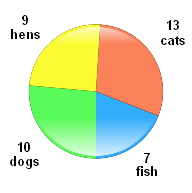HOME MATH DICTIONARY DOWNLOAD FEEDBACK DISCLAIMER
 Question: What is Pie chart ? Answer: A pie chart is a circular chart which is divided into sectors, illustrating relative magnitudes or frequencies or percents. In a pie chart, the arc length of each sector is proportional to the quantity it represents. Together, the sectors create a full disk. A pie chart, like a pictogram, is a way of presenting statistical data in a visual form which can be easily understood. A circle is divided up, like a pie, into sectors in proportion to the frequencies of the data.